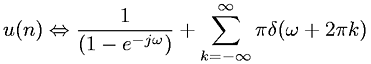Equations > Signal Processing > Fourier Transform Pairs > Discrete-Time Fourier transform of unit step function

### Discrete-Time Fourier transform of unit step functionLatex Code:

MathML Code:

 $u\left(n\right)⇔\frac1\left(1-{e}^{-j\omega }\right)+\sum _{k=-\infty }^{\infty }\pi \delta \left(\omega +2\pi k\right)$

MathType 5.0: